# 8 8 Solving Radical Equations and Inequalities Objective

• Slides: 33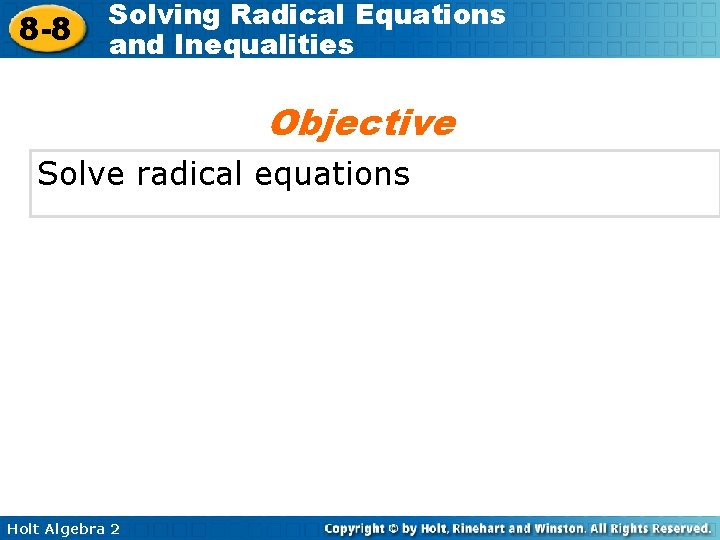8 -8 Solving Radical Equations and Inequalities Objective Solve radical equations Holt Algebra 2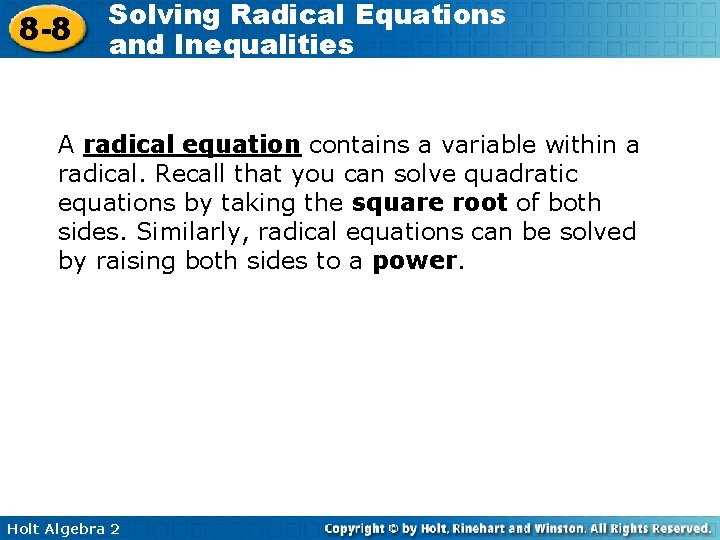8 -8 Solving Radical Equations and Inequalities A radical equation contains a variable within a radical. Recall that you can solve quadratic equations by taking the square root of both sides. Similarly, radical equations can be solved by raising both sides to a power. Holt Algebra 2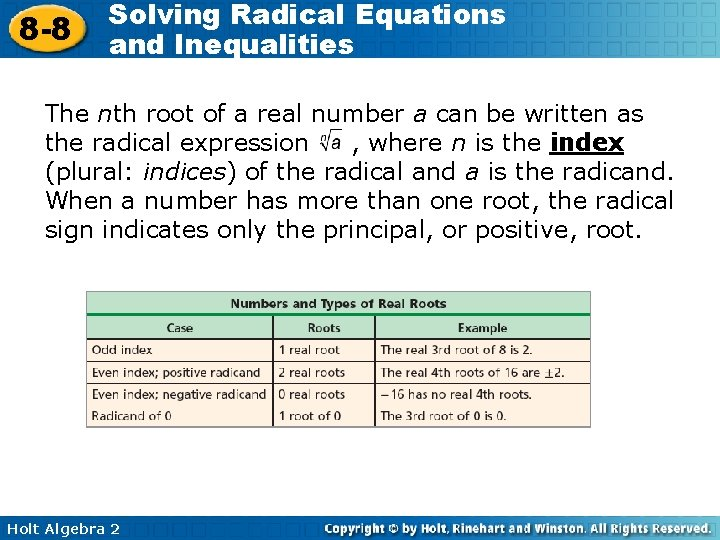8 -8 Solving Radical Equations and Inequalities The nth root of a real number a can be written as the radical expression , where n is the index (plural: indices) of the radical and a is the radicand. When a number has more than one root, the radical sign indicates only the principal, or positive, root. Holt Algebra 2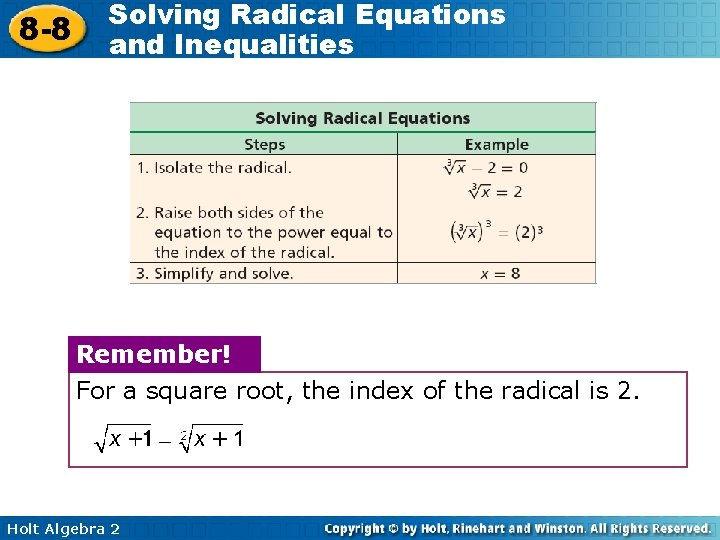8 -8 Solving Radical Equations and Inequalities Remember! For a square root, the index of the radical is 2. Holt Algebra 28 -8 Solving Radical Equations and Inequalities Example 1 A: Solving Equations Containing One Radical Solve each equation. Check Subtract 5. Simplify. Square both sides. Simplify. Solve for x. Holt Algebra 2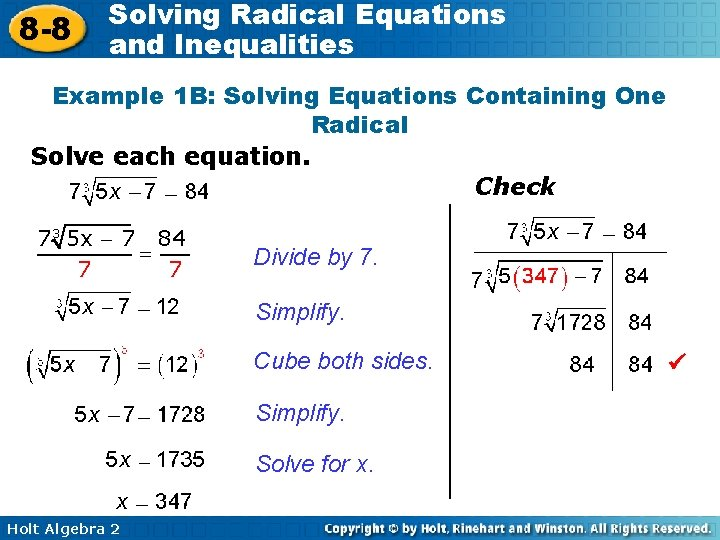8 -8 Solving Radical Equations and Inequalities Example 1 B: Solving Equations Containing One Radical Solve each equation. Check 7 3 5 x - 7 84 = 7 7 Divide by 7. 7 Simplify. Cube both sides. Simplify. Solve for x. Holt Algebra 28 -8 Solving Radical Equations and Inequalities Check It Out! Example 1 a Solve the equation. Check Subtract 4. Simplify. Square both sides. Simplify. Solve for x. Holt Algebra 2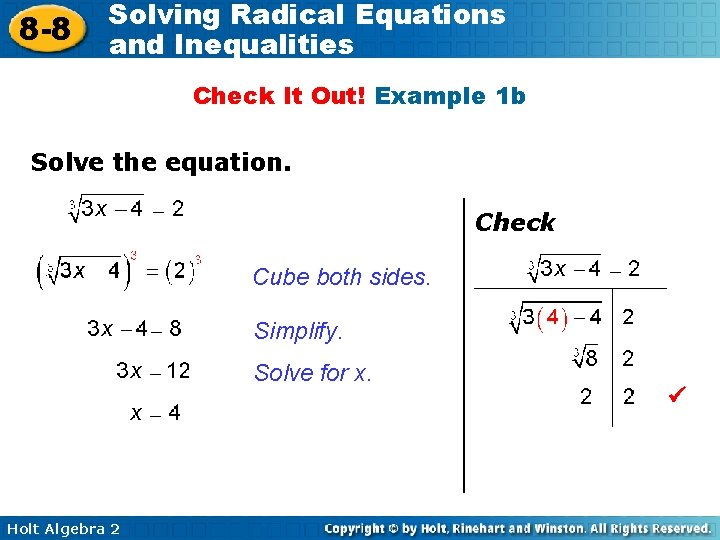8 -8 Solving Radical Equations and Inequalities Check It Out! Example 1 b Solve the equation. Check Cube both sides. Simplify. Solve for x. Holt Algebra 28 -8 Solving Radical Equations and Inequalities Check It Out! Example 1 c Solve the equation. Check Divide by 6. Square both sides. Simplify. Solve for x. Holt Algebra 2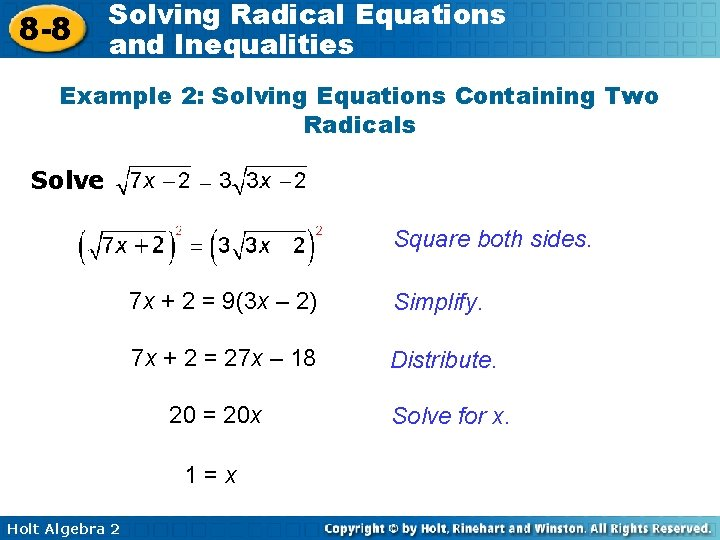8 -8 Solving Radical Equations and Inequalities Example 2: Solving Equations Containing Two Radicals Solve Square both sides. 7 x + 2 = 9(3 x – 2) Simplify. 7 x + 2 = 27 x – 18 Distribute. 20 = 20 x 1=x Holt Algebra 2 Solve for x.8 -8 Solving Radical Equations and Inequalities Example 2 Continued Check 3 Holt Algebra 2 3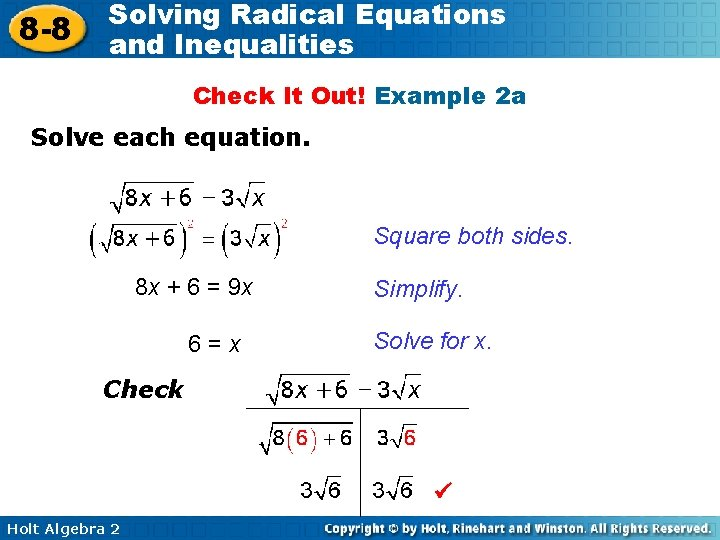8 -8 Solving Radical Equations and Inequalities Check It Out! Example 2 a Solve each equation. Square both sides. 8 x + 6 = 9 x 6=x Simplify. Solve for x. Check Holt Algebra 2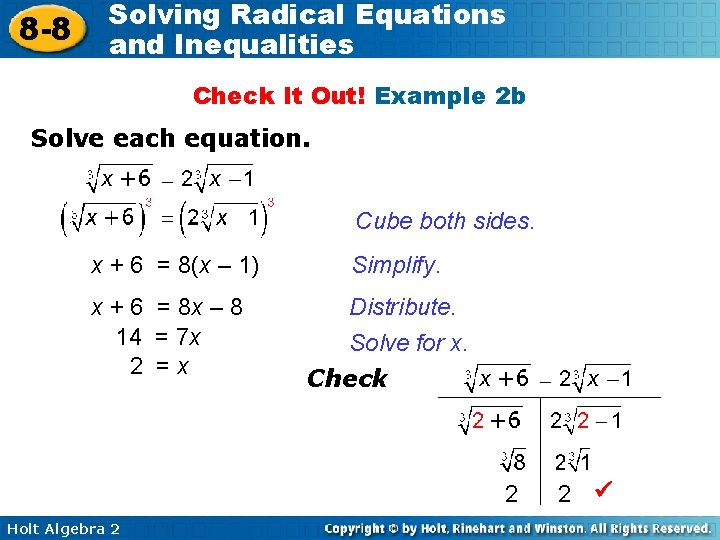8 -8 Solving Radical Equations and Inequalities Check It Out! Example 2 b Solve each equation. Cube both sides. x + 6 = 8(x – 1) Simplify. x + 6 = 8 x – 8 14 = 7 x 2 =x Distribute. Solve for x. Check 2 Holt Algebra 2 2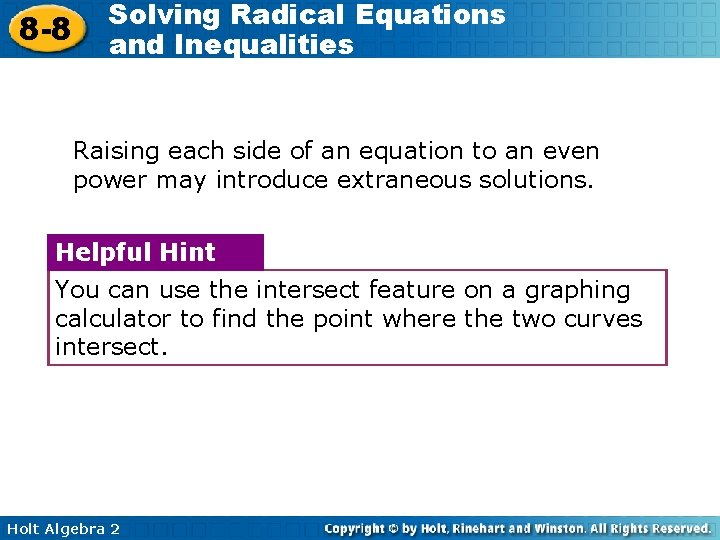8 -8 Solving Radical Equations and Inequalities Raising each side of an equation to an even power may introduce extraneous solutions. Helpful Hint You can use the intersect feature on a graphing calculator to find the point where the two curves intersect. Holt Algebra 2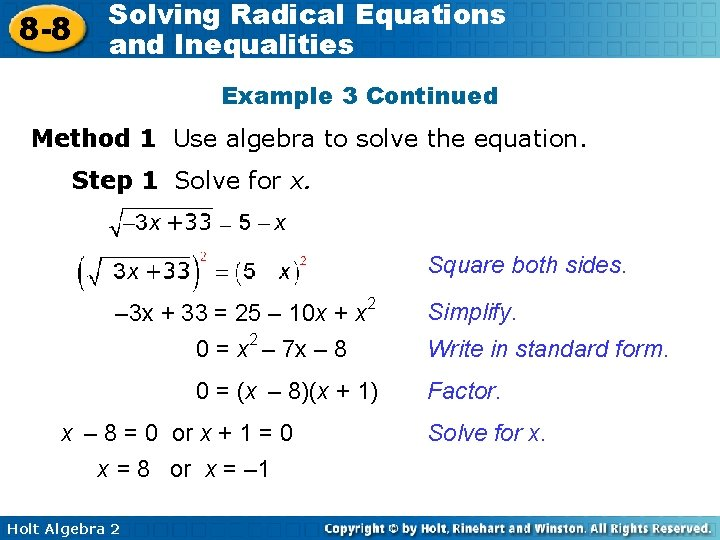8 -8 Solving Radical Equations and Inequalities Example 3 Continued Method 1 Use algebra to solve the equation. Step 1 Solve for x. Square both sides. – 3 x + 33 = 25 – 10 x + x 2 0 = x 2 – 7 x – 8 0 = (x – 8)(x + 1) x – 8 = 0 or x + 1 = 0 x = 8 or x = – 1 Holt Algebra 2 Simplify. Write in standard form. Factor. Solve for x.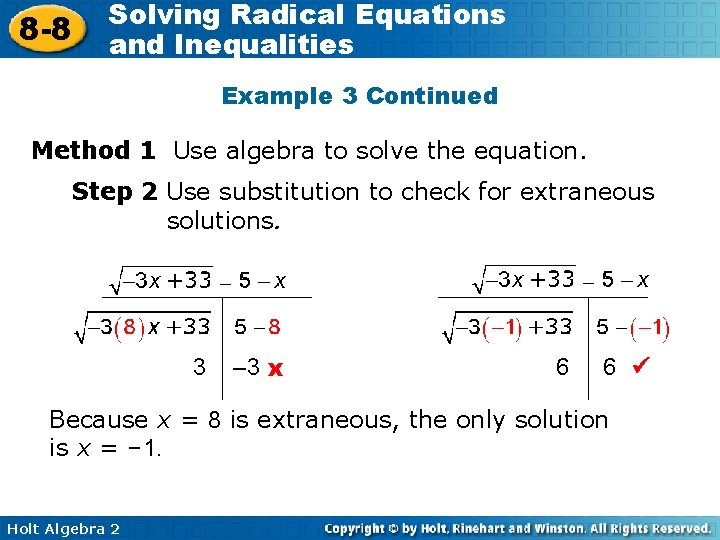8 -8 Solving Radical Equations and Inequalities Example 3 Continued Method 1 Use algebra to solve the equation. Step 2 Use substitution to check for extraneous solutions. 3 – 3 x 6 6 Because x = 8 is extraneous, the only solution is x = – 1. Holt Algebra 2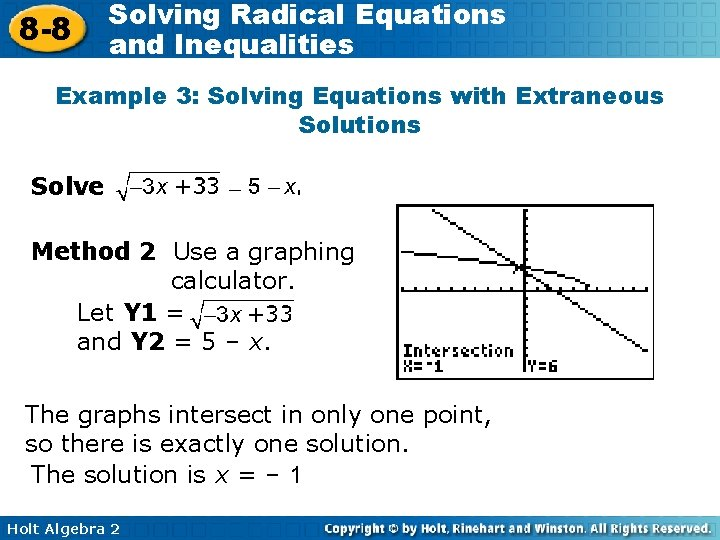8 -8 Solving Radical Equations and Inequalities Example 3: Solving Equations with Extraneous Solutions Solve . Method 2 Use a graphing calculator. Let Y 1 = and Y 2 = 5 – x. The graphs intersect in only one point, so there is exactly one solution. The solution is x = – 1 Holt Algebra 2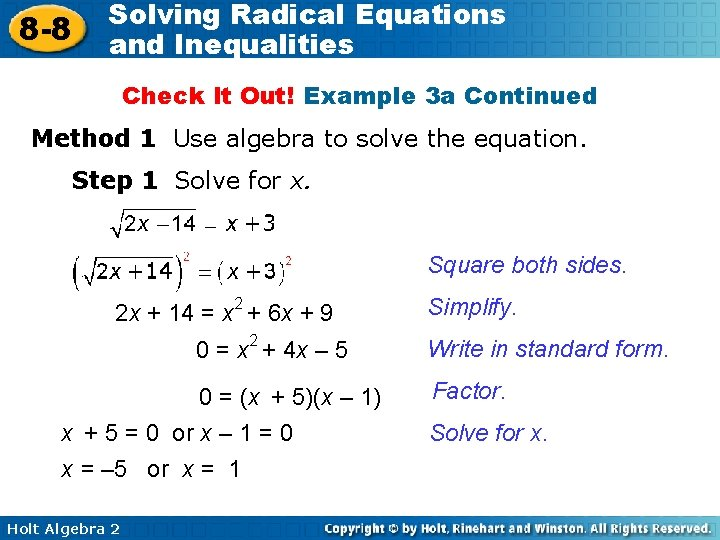8 -8 Solving Radical Equations and Inequalities Check It Out! Example 3 a Continued Method 1 Use algebra to solve the equation. Step 1 Solve for x. Square both sides. 2 x + 14 = x 2 + 6 x + 9 0 = x 2 + 4 x – 5 0 = (x + 5)(x – 1) x + 5 = 0 or x – 1 = 0 x = – 5 or x = 1 Holt Algebra 2 Simplify. Write in standard form. Factor. Solve for x.8 -8 Solving Radical Equations and Inequalities Check It Out! Example 3 a Continued Method 1 Use algebra to solve the equation. Step 2 Use substitution to check for extraneous solutions. 2 – 2 x 4 4 Because x = – 5 is extraneous, the only solution is x = 1. Holt Algebra 2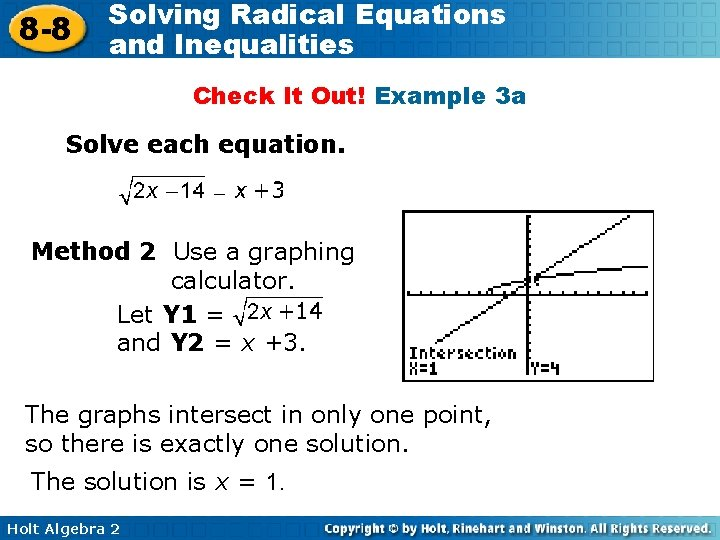8 -8 Solving Radical Equations and Inequalities Check It Out! Example 3 a Solve each equation. Method 2 Use a graphing calculator. Let Y 1 = and Y 2 = x +3. The graphs intersect in only one point, so there is exactly one solution. The solution is x = 1. Holt Algebra 2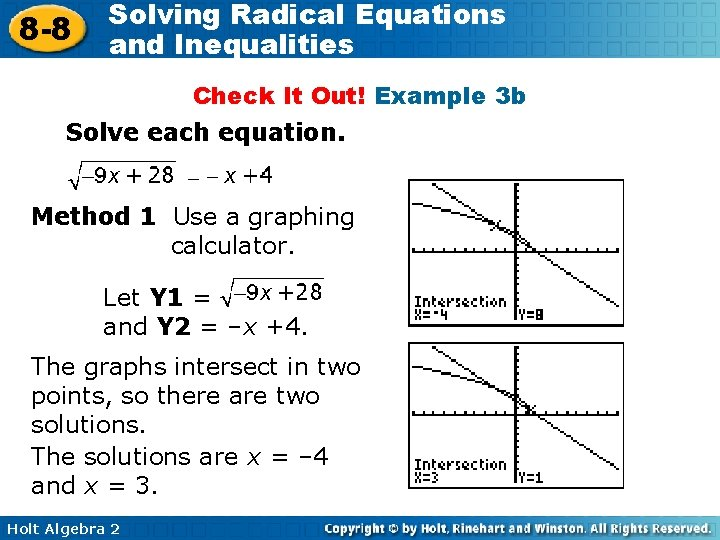8 -8 Solving Radical Equations and Inequalities Check It Out! Example 3 b Solve each equation. Method 1 Use a graphing calculator. Let Y 1 = and Y 2 = –x +4. The graphs intersect in two points, so there are two solutions. The solutions are x = – 4 and x = 3. Holt Algebra 2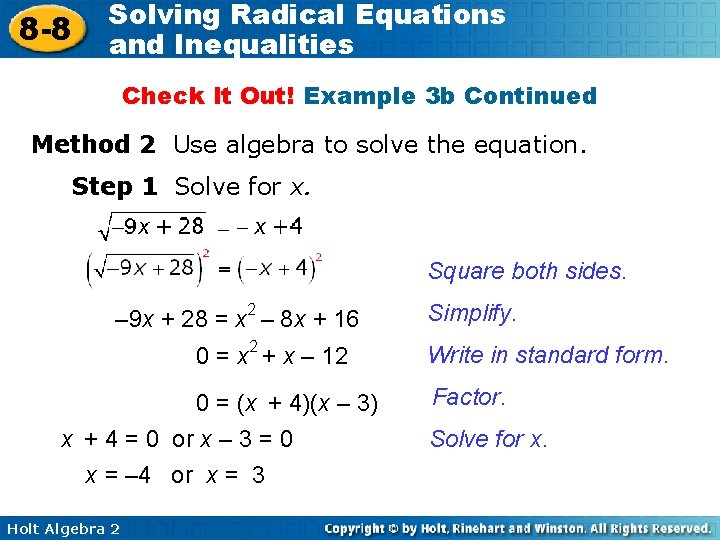8 -8 Solving Radical Equations and Inequalities Check It Out! Example 3 b Continued Method 2 Use algebra to solve the equation. Step 1 Solve for x. Square both sides. – 9 x + 28 = x 2 – 8 x + 16 0 = x 2 + x – 12 0 = (x + 4)(x – 3) x + 4 = 0 or x – 3 = 0 x = – 4 or x = 3 Holt Algebra 2 Simplify. Write in standard form. Factor. Solve for x.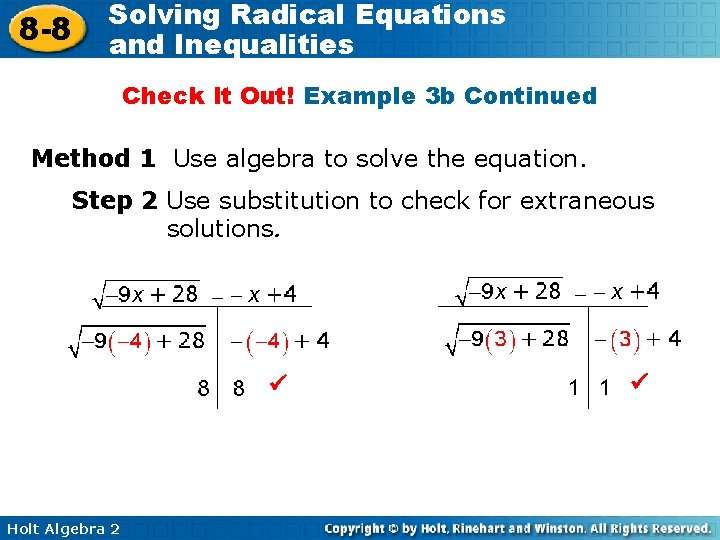8 -8 Solving Radical Equations and Inequalities Check It Out! Example 3 b Continued Method 1 Use algebra to solve the equation. Step 2 Use substitution to check for extraneous solutions. Holt Algebra 2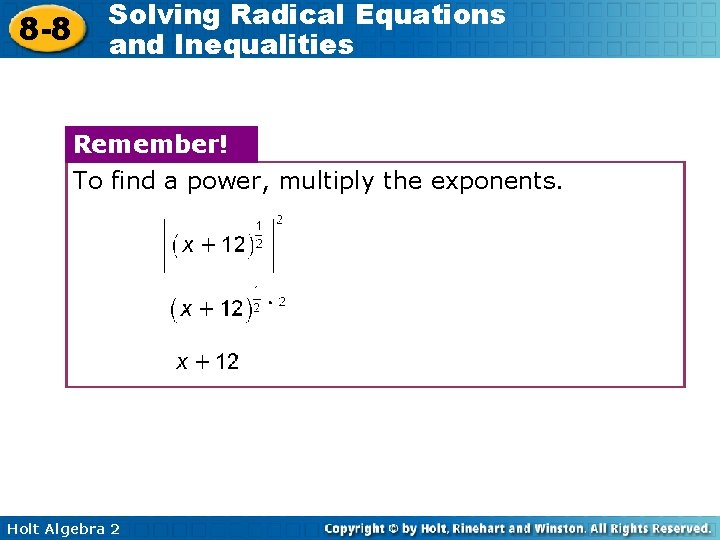8 -8 Solving Radical Equations and Inequalities Remember! To find a power, multiply the exponents. Holt Algebra 2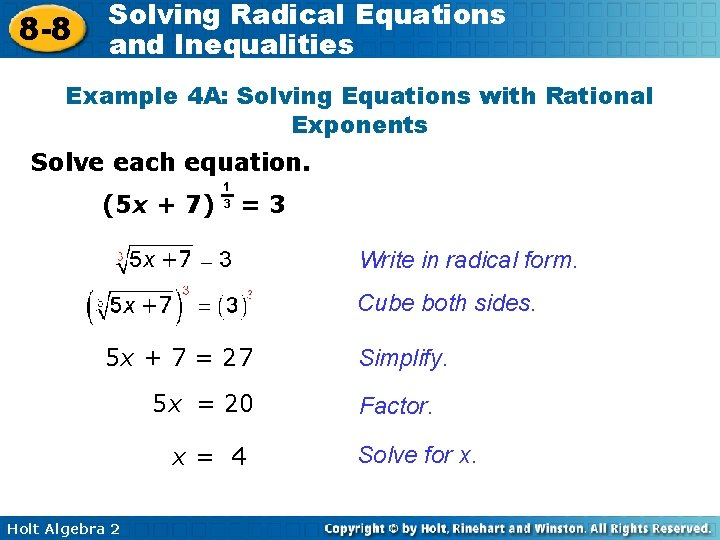8 -8 Solving Radical Equations and Inequalities Example 4 A: Solving Equations with Rational Exponents Solve each equation. (5 x + 7) 1 3 =3 Write in radical form. Cube both sides. 5 x + 7 = 27 5 x = 20 x= 4 Holt Algebra 2 Simplify. Factor. Solve for x.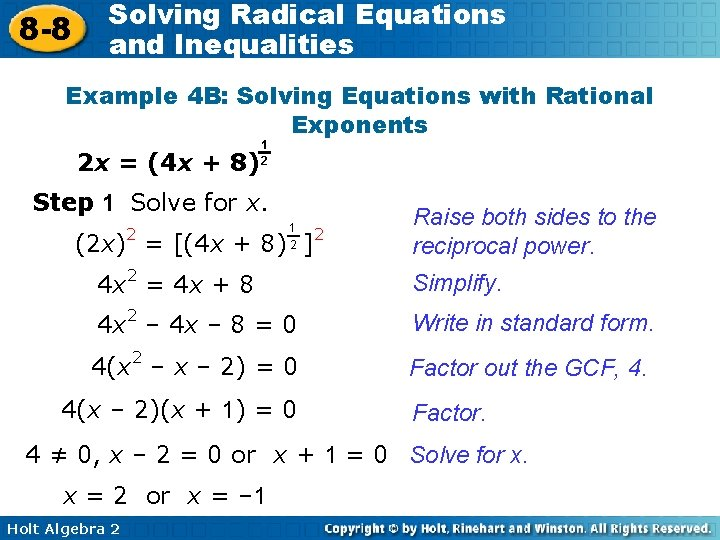8 -8 Solving Radical Equations and Inequalities Example 4 B: Solving Equations with Rational Exponents 2 x = (4 x + 8) 1 2 Step 1 Solve for x. 1 2 (2 x)2 = [(4 x + 8) ]2 Raise both sides to the reciprocal power. 4 x 2 = 4 x + 8 Simplify. 4 x 2 – 4 x – 8 = 0 Write in standard form. 4(x 2 – x – 2) = 0 Factor out the GCF, 4. 4(x – 2)(x + 1) = 0 Factor. 4 ≠ 0, x – 2 = 0 or x + 1 = 0 Solve for x. x = 2 or x = – 1 Holt Algebra 2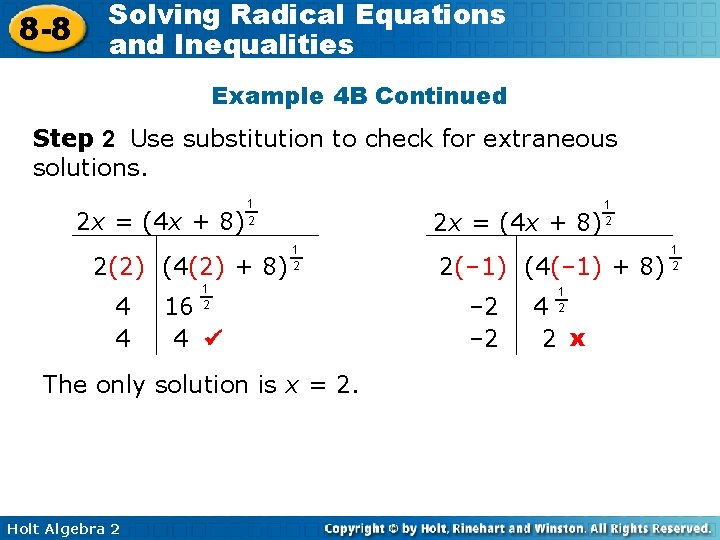8 -8 Solving Radical Equations and Inequalities Example 4 B Continued Step 2 Use substitution to check for extraneous solutions. 2 x = (4 x + 8) 1 2 2(2) (4(2) + 8) 4 4 2 x = (4 x + 8) 1 2 16 4 The only solution is x = 2. Holt Algebra 2 1 2 2(– 1) (4(– 1) + 8) – 2 1 2 4 2 x 1 28 -8 Solving Radical Equations and Inequalities Check It Out! Example 4 a Solve each equation. (x + 5) 1 3 =3 Write in radical form. Cube both sides. x + 5 = 27 x = 22 Holt Algebra 2 Simplify. Solve for x.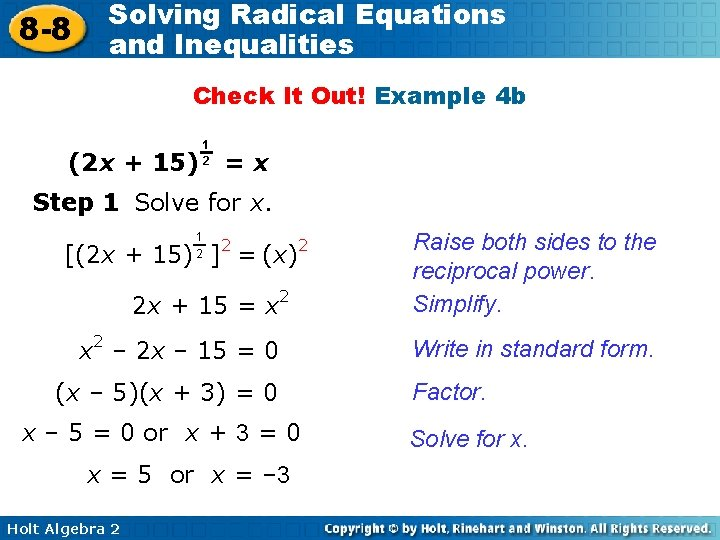8 -8 Solving Radical Equations and Inequalities Check It Out! Example 4 b (2 x + 15) 1 2 =x Step 1 Solve for x. 1 2 2 [(2 x + 15) ] = (x) 2 2 x + 15 = x 2 – 2 x – 15 = 0 (x – 5)(x + 3) = 0 x – 5 = 0 or x + 3 = 0 x = 5 or x = – 3 Holt Algebra 2 Raise both sides to the reciprocal power. Simplify. Write in standard form. Factor. Solve for x.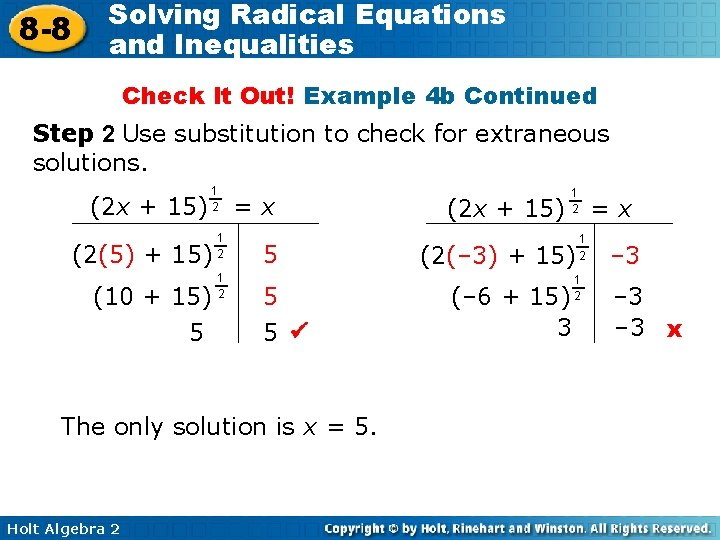8 -8 Solving Radical Equations and Inequalities Check It Out! Example 4 b Continued Step 2 Use substitution to check for extraneous solutions. (2 x + 15) 1 2 (2(5) + 15) (10 + 15) 5 1 2 =x 5 5 5 The only solution is x = 5. Holt Algebra 2 (2 x + 15) 1 2 (2(– 3) + 15) (– 6 + 15) 3 =x 1 2 – 3 – 3 x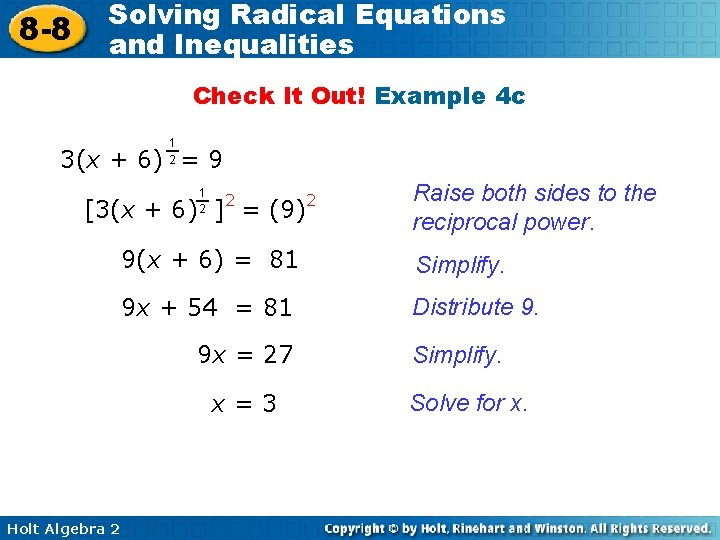8 -8 Solving Radical Equations and Inequalities Check It Out! Example 4 c 1 2 3(x + 6) = 9 1 2 2 [3(x + 6) ] = (9) Raise both sides to the reciprocal power. 9(x + 6) = 81 Simplify. 9 x + 54 = 81 Distribute 9. 9 x = 27 x=3 Holt Algebra 2 2 Simplify. Solve for x.8 -8 Solving Radical Equations and Inequalities Check It Out! Example 6 The speed s in miles per hour that a car is traveling when it goes into a skid can be estimated by using the formula s = 30 fd , where f is the coefficient of friction and d is the length of the skid marks in feet. A car skids to a stop on a street with a speed limit of 30 mi/h. The skid marks measure 35 ft, and the coefficient of friction was 0. 7. Was the car speeding? Explain. Use the formula to determine the greatest possible length of the driver’s skid marks if he was traveling 30 mi/h. Holt Algebra 2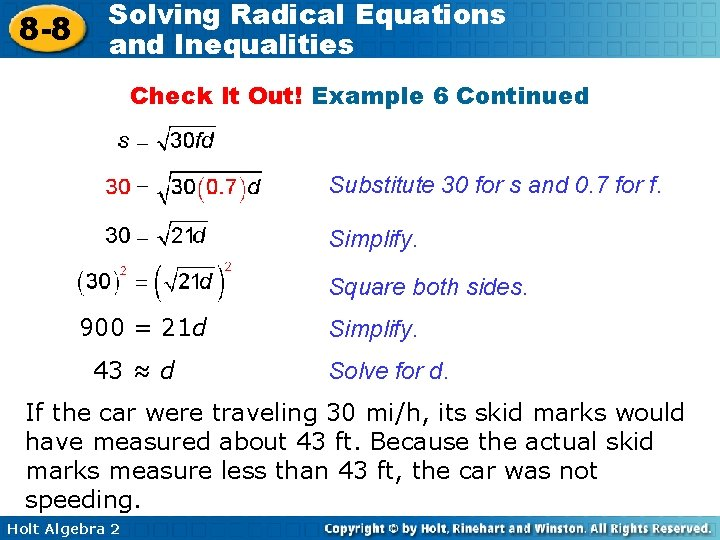8 -8 Solving Radical Equations and Inequalities Check It Out! Example 6 Continued Substitute 30 for s and 0. 7 for f. Simplify. Square both sides. 900 = 21 d 43 ≈ d Simplify. Solve for d. If the car were traveling 30 mi/h, its skid marks would have measured about 43 ft. Because the actual skid marks measure less than 43 ft, the car was not speeding. Holt Algebra 2